Science Journal of Applied Mathematics and Statistics
Volume 4, Issue 2, April 2016, Pages: 64-73

Review Article

Modeling and Forecasting Kenyan GDP Using Autoregressive Integrated Moving Average (ARIMA) Models

Musundi Sammy Wabomba*, M’mukiira Peter Mutwiri, Mungai Fredrick

Department of Physical Sciences, Chuka University, Nairobi, Kenya(Musundi S. W.)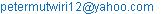(M’mukiira P. T.)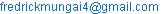(Mungai F.)

*Corresponding author

Musundi Sammy Wabomba, M’mukiira Peter Mutwiri, Mungai Fredrick. Modeling and Forecasting Kenyan GDP Using Autoregressive Integrated Moving Average (ARIMA) Models. Science Journal of Applied Mathematics and Statistics. Vol. 4, No. 2, 2016, pp. 64-73. doi: 10.11648/j.sjams.20160402.18

Received: March 14, 2016; Accepted: March 25, 2016; Published: April 13, 2016

Abstract: The Gross Domestic Product (GDP) is the market value of all goods and services produced within the borders of a nation in a year. In this paper, Kenya’s annual GDP data obtained from the Kenya National Bureau of statistics for the years 1960 to 2012 was studied. Gretl and SPSS 21 statistical softwares were used to build a class of ARIMA (autoregressive integrated moving average) models following the Box-Jenkins method to model the GDP. ARIMA (2, 2, 2) time series model was established as the best for modeling the Kenyan GDP according to the recognition rules and stationary test of time series under the AIC criterion. The results of an in-sample forecast showed that the relative and predicted values were within the range of 5%, and the forecasting effect of this model was relatively adequate and efficient in modeling the annual returns of the Kenyan GDP. Finally, we used the fitted ARIMA model to forecast the GDP of Kenya for the next five years.

Keywords: Gross Domestic Product (GDP), Gretl and SPSS 21 Statistical Softwares, ARIMA (Autoregressive Integrated Moving Average) Models, AIC Criterion

Contents

1. Introduction

As an aggregate measure of total economic production for a country, GDP represents the market value of all goods and services produced by the economy during the period measured, including personal consumption, government purchases, private inventories, paid-in construction costs and the foreign trade balance (exports are added, imports are subtracted). It is an area of key interest for most researchers in the field of business in general and of economics in particular. The issue of GDP has become the biggest concern amongst macro economy variables. Data on GDP is regarded as an important index for assessing the national economic development and for judging the operating status of macro economy as a whole .

GDP is the aggregate statistic of all economic activity and captures a broader coverage of the economy than other macro-economic variables. It is the market value of all final goods and services produced within the borders of a nation in a year. It is often considered the best measure of how well the economy is performing. GDP can be measured in three ways. First, the Expenditure approach, it consists of household, business and government purchases of goods and services and net exports. Second, the Production approach, it is equal to the sum of the value added at every stage of production (the intermediate stages) by all industries within the country, plus taxes and fewer subsidies on products in the period. Third is the Income approach, it is equal to the sum of all factor income generated by production in the country (the sum of remuneration of employees, capital income, and gross operating surplus of enterprises i.e. profit, taxes on production and imports less subsidies) in a period .

Besides these, it is also a vital basis for government to set up economic developmental strategies and policies. Therefore, an accurate prediction of GDP is necessary to get an insightful idea of future trend of an economy. Raw historical and current data on GDP cannot be used to frame suitable economic development strategies, economic policies and allocation of funds on different priorities for government as well as individual firms in a particular industry. It needs a reliable estimate of GDP in some period ahead, which is only possible by forecasting GDP as accurately as possible using a suitable time series model. However it is not easy to identify the exact variables that affect the GDP.

2. Literature Review

We provide both theoretical and empirical literature on GDP process and its forecasting. The coverage is organized into three sections. Section 2.1 is on theoretical literature review, section 2.2 is on ARIMA models while section 2.3 is devoted to presentation of empirical literature review; these three sections are further discussed in subsections.

2.1. GDP

Economic growth is measured in terms of an increase in the size of a nation's economy. A broad measure of an economy's size is its output. The most widely-used measure of economic output is the GDP. The three basic ways to determine a nation’s GDP are; the Expenditure approach, the Production approach and the Income approach.

The Expenditure Approach of determining GDP adds up the market value of all domestic expenditures made on final goods and services in a single year, including consumption expenditures, investment expenditures, government expenditures, and net exports. Add all of the expenditures together and you determine GDP.

The Production approach, also called the Net Product or Value added method requires three stages of analysis. First gross value of output from all sectors is estimated. Then, intermediate consumption such as cost of materials, supplies and services used in production final output is derived. Then gross output is reduced by intermediate consumption to develop net production.

The Income Approach of determining GDP is to add up all the income earned by households and firms in the year. The total expenditures on all of the final goods and services are also income received as wages, profits, rents, and interest income. GDP is determined by adding together all of the wages, profits, rents, and interest income.

The three methods of measuring GDP should result in the same number, with some possible difference caused by statistical and rounding differences. The credibility of data is always a significant concern in any form of research. An advantage of using the Expenditure Method is data integrity. The source data for expenditure components is considered to be more reliable than for either income or production components.

GDP as examined using the Expenditure Approach is reported as the sum of four components . The formula for determining GDP is:

C + I + G + (X - M) = GDP          (1)

Where:

C = Personal Consumption Expenditures

I = Gross Private Fixed Investment

G = Government Expenditures and Investment

X = Net Exports

M = Net Imports

2.1.1. Government Expenditure

 studied the effect of the size of government expenditure on economic growth for 115 countries for the 1960-1980 periods. He found that although a higher rate of increase in government expense is associated with a higher growth rate a higher share of government expenditure in GDP dampens growth. In his studies,  considers government to be complimentary, not a substitute, for private investment, and examines the effect of government expenditures on growth in this light. He found that an increase in government expenditure led to the increase in GDP.

 examined an endogenous growth model that suggests a possible relationship between the share of government spending in GDP and the growth rate of per capita real GDP. The key feature of the model by  is the presence of constant returns to capital that broadly includes private capital and public services. To the extent that public services are considered an input to production, a possible linkage arises between the size of government and economic growth.

2.1.2. Inflation Rate and GDP

In , it is essential to study inflation in each country because inflation is devastating. Inflation created problem and introduced noises in the functioning of the economy that is likely to affect economic growth. However it is not an easy task to tackle the inflation problem effectively. In order to handle inflation problem successfully, accurate assessment of the causes of the problem is critical as strong diagnosis of the nature of the problem will lead to the application of inappropriate cures that might produce unintended adverse effect on the economy.

 studied that in the history of inflationary in Malaysia, 1973 and 1974 were exceptional years. Inflation rose significantly in both the international and domestic market in 1973.The sharp oil price increase in 1973 and 1974 was the principal reason for the escalation of world inflation in 1973-1974. However, the effect of an increase in oil price was actually felt in 1974. The substantial price increase in 1973 were bought about the mainly of the shortages of food and raw material arising from bad weather and increased an aggregate demand.

Consequently consumer price in Malaysia began to rise and had reach of high level of 10.62 percent by the end of the year 1973. In 1974, the surge in the oil price by over 230 percent put strong fuel of inflation and the inflation rate in Malaysia was increased to its record high of 17.29 percent. A year later Malaysian economy slumped into its great recession with GDP growth rate of only 0.8 percent in 1975 compared to 8.3 percent in 1974.

The inflation rate in Malaysia was last reported at 2 percent in November of 2010. From 2005 until 2010, the average inflation rate in Malaysia was 2.77 percent reaching an historical high of 8.50 percent in July of 2008 and a record low of -2.40 percent in July of 2009. Inflation rate refers to a general rise in prices measured against a standard level of purchasing power. The most known measures of Inflation are the CPI which measures consumer prices, and the GDP deflator, which measures inflation in the whole of the domestic economy.

2.1.3. Export

The study by  supports the view that export growth promotes overall economic growth. A serious drawback of cross section studies, however, is that the issue of causality between export growth and GDP growth is not address directly. However, faster growing economics may give rise to a greater dynamic export. Many authors have doubted the validity conclusions based on cross country studies. Sheehey (1990), for example investigates whether there are other productive categories besides export whose growth has a similar relationship to GDP. Studies have found that a number of other determinant factors contribute to economic growth.

 studied the economic success of new industrial countries such as Indonesia, Malaysia, Philippines, Singapore and Thailand using time data series from the year 1966 until 1998 to find out whether export is the cause of the countries’ economic growth. They found that the link between export and economic growth lies in the development policy. Interestingly, their studies also found that it is economic development that causes economic growth, and not vice versa.

Using the approach by  of defining GDP net of exports, he found weak support for exports as an engine of growth and very little evidence consistent with a government-led growth hypothesis.  found very weak support for the contention that export growth promotes GDP growth. Support for the alternate contention that GDP growth promotes export growth was also weak, although somewhat stronger than the former.

A number of studies have found that export growth exerts a positive impact on GDP growth in less developed countries (LDCs), even when capital and labor are controlled for. Using a similar framework but recognizing the possible heterogeneity of exports, the present paper finds, for the 1960–1980 period, that while the primary export sector exhibits little or no effect on GDP growth in LCDs, there is a differential positive impact by the manufacturing export sector.

Studies by  used co-integration analysis and the causality approach by Johansen and ECM to analyze the relationship between consumption expenditure and economic growth. The study concludes that government expenditure may have a role as a catalyst and complement determinant factors to economic growth in Malaysia.

Meanwhile,  studied the relationship between per capita saving and per capita GDP in India using the Granger causality test based on the Toda and Yamamoto approach. The data used were from 1950 to 2004. The types of savings include household, corporate and public savings. The results of their studies showed that there are no causal relationships between per capita GDP with per capita household savings or per capita corporate savings coming from any direction. However, there exists a bilateral causal relationship between per capita household savings and per capita corporate savings.

 tried to observe the causal relationship between electricity usage and economic growth amongst four ASEAN countries namely Indonesia, Malaysia, Singapore, and Thailand using modern time series data for the years 1971 to 2002. They found that there is a bilateral causal relationship between electricity use and economic growth in Malaysia and Singapore, while a one-way causal relationship exists towards economic growth through electricity usage in Indonesia and Thailand.

2.1.4. GDP Forecasting

Econometric forecasting involves the application of both statistical and mathematical models to predict future developments in the economy. It allows economists to review past economic trends and forecast how recent economic changes will alter the patterns of past trends.

A time series data of GDP consists of observations generated successively over time. Such data are ordered with respect to time and successive observations may be dependent. The observed time series is generally referred to as time series realization of an underlying process. The data may indicate that there is a trend over time, which is a long term behavior underlying the data. The trend may either be increasing, decreasing, or even constant.

There may be a cyclical fluctuation, which is a pattern of ups and downs over time. Also, the data may show that the underlying process has periodic fluctuations of constant length, which is seasonal behavior. Modeling therefore, captures this underlying process using the observed time series so that one can forecast what would be the likely realization at a time point in future.

In forecasting macroeconomic time series variables like GDP, one has many possible types of models to choose from: vector error correction models, autoregressive conditional heteroskedasticity (ARCH)-based models, or various possible combinations. However, ARIMA models have proven themselves to be relatively robust especially when generating short-run GDP forecasts and have frequently outperformed more sophisticated structural models in terms of short-run forecasting ability [20,13].

2.2. Auto-regressive Integrated Moving Average (ARIMA) Models

Autoregressive Integrated Moving Average models (ARIMA models) were popularized by George Box and Gwilym Jenkins in the early 1970s. It’s an iterative process that involves four stages; identification, estimation, diagnostic checking and forecasting of time series.

According to , ARIMA models are a class of linear models that is capable of representing stationary as well as non-stationary. They do not involve independent variables in their construction, but rather make use of the information in the series itself to generate forecasts. ARIMA models therefore, rely heavily on autocorrelation patterns in the data.

ARIMA methodology of forecasting is different from most methods because it does not assume any particular pattern in the historical data of the series to be forecast. It uses an interactive approach of identifying a possible model from a general class of models. The chosen model is then checked against the historical data to see if it accurately describes the series. Most of the traditional forecasting models therefore, provide a limited number of models relative to the complex behaviour of many time series with little guidelines and statistical tests for verifying the validity of the selected model.

2.2.1. Moving Average (MA) Process

This is a time series model which uses past errors as explanatory variable . Let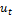(t=1,2,3,...) be a white noise process, a sequence of independently and identically distributed (iid) random variables with E()=0 and Var() =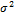. Then the qth order MA model is given as: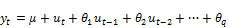(2)

This model is expressed in terms of past errors and thus we estimate the coefficients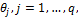and use the model for forecasting. Therefore only q errors will affect the current level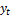but higher order errors do not affect. This implies that it is a short memory model.

2.2.2. Auto-Regression (AR)

According to , an autoregressive model of order p, an AR (p) can be expressed as;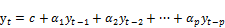(3)

Where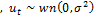.

The model is expressed in terms of past values and therefore, we wish to estimate the coefficients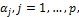and use the model for forecasting. In this case, all previous values will have cumulative effects on the current leveland thus, it is a long-run memory model. The ACF(s) therefore does not die out easily since it takes a longer time to have ACF close to zero.

Partial Autocorrelation Functions (PACF) measures the correlation between an observation k periods ago and the current observation, after controlling for observations at intermediate lags (i.e. all lags <k).

PACF (k) = ACF (k) after controlling the effects of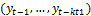. Thus PACF (k) can be found as the coefficient of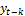in the regression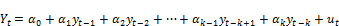(4)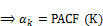Hence the PACF is useful for telling the maximum order of an AR process.

Auto-regressive (AR) models can be coupled with moving average (MA) models to form a general and useful class of time series models called Autoregressive Moving Average (ARMA) models. These can be used when the data are stationary.

2.2.3. Autoregressive Moving Average Model (ARMA)

 expressed an ARMA (p, q) model as follows: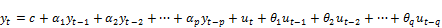(5)

This is a combination of both AR and MA models. In this case therefore, neither ACF nor PACF can solely provide the information on the maximum orders of p or q.

This class of models can further be extended to non-stationary series by allowing the differencing of the data series resulting to Autoregressive Integrated Moving Average (ARIMA) models.

2.2.4. Autoregressive Integrated Moving Average (ARIMA) Process

There are a large variety of ARIMA models . The general non-seasonal model is known as ARIMA (p, d, q): where p is the number of autoregressive terms, d is the number of differences and q is the number of moving average terms. A white noise model is classified as ARIMA (0, 0, 0) since there exists no AR part becausedoes not depend on yt-1, there is no differencing involved and also there’s no MA part sincedoes not depend on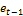.

For instance, ifis non-stationary, we take a first-difference ofso that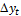becomes stationary.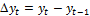(d = 1 implies one time differencing)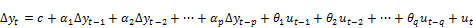(6)

is an ARIMA (p, 1, q) model.

A random walk model is classified as ARIMA (0, 1, 0) because there is no AR and MA part involved and only one difference exists.

2.3. Conceptual Framework of Box Jenkins Methodology

According to , the process uses four iterative stages of Modeling that involves; identification, estimation, diagnostic checking and forecasting (See figure 1 below).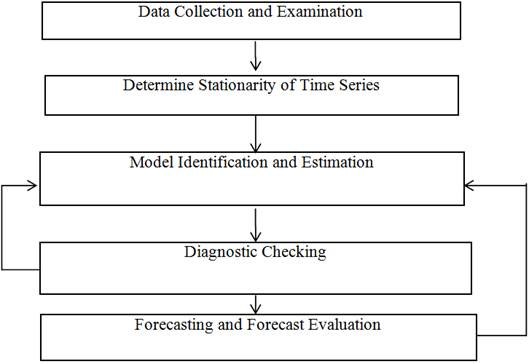Figure 1. ARIMA forecasting procedure.

2.3.1. Model Identification

A preliminary Box-Jenkins analysis with a plot of the initial data should be run as the starting point in determining an appropriate model. The input data must be adjusted to form a stationary series and identify seasonality in the dependent series (seasonally differencing it if necessary), and using plots of the autocorrelation and partial autocorrelation functions of the dependent time series to decide which (if any) autoregressive (AR) or moving average (MA) component should be used in the model.

2.3.2. Model Estimation

The parameters of the selected ARIMA (p, d, q) model can be estimated consistently by least-squares or by maximum likelihood. Both estimation procedures are based on the computation of the innovations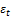from the values of the stationary variable. The least-squares methods minimize the sum of squares;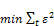(7)

The log-likelihood can be derived from the joint probability density function of the innovations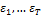, that takes the following form under the normality assumption,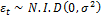: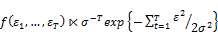(8)

In order to solve the estimation problem, equations 6 and 7 should be written in terms of the observed data and the set of parameters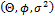. An ARMA (p, q) process for the stationary transformation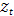can be expressed as: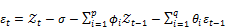(9)

Then, to compute the innovations corresponding to a given set of observations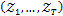and parameters, it is necessary to count with the starting values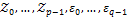. More realistically, the innovations should be approximated by setting appropriate conditions about the initial values, giving to conditional least squares or conditional maximum likelihood estimators.

2.3.3. Diagnostic Checking

Before using the model for forecasting, it must be checked for adequacy (diagnostic checking). The model is considered adequate if the residuals left over after fitting the model is simply white noise and also the pattern of ACF and PACF of the residuals may suggest how the model can be improved.

Akaike’s Information Criterion (AIC) is one of the most robust methods used in estimating parameters of an identified model.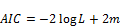(10)

Where; L denotes the likelihood and m is the number of parameters estimated in the model such that;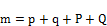(11)

However, not all computer programs produce the AIC or the likelihood L, thus it is not always possible to find the AIC for a given model. A useful approximation to the AIC is therefore denoted as;(12)

As an alternative to AIC, the Bayesian Information Criteria (BIC) and the Schwarz- Bayesian Information Criteria (SBC) are also used as model diagnostics. The SBC is given by;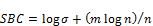(13)

2.3.4. Model Forecasting

Model forecasting states the difference between in-sample forecasting and out-of sample forecasting. In-sample forecasting for instance, explains how the chosen model fits the data in a given sample while Out-of-sample forecasting on the other hand, is concerned with determining how a fitted model forecasts future values of the regressand, given the values of the regressors.

To build a reliable model, the following factors are highly considered in forecasting;

a) The level of accuracy required – forecasts should be prepared as accurately as possible to facilitate the decision making process especially made on the basis of the GDP forecasts.

b) Availability of data and information – a wealth of reliable and up-to-date GDP data results to a reliable model.

c) The time horizon that the GDP forecast is intended to cover. This study for instance, covered a short run period.

3. Methodology

3.1. Research Design

The research design was experimental, since the main objective of this study was to determine or forecast the GDP level in Kenya. Experimental research allows the researcher to control the situation and identify the cause and effect relationships between variables and also distinguish placebo effects from treatment effects. According to , experimental research is often used where there is time priority in a causal relationship (cause precedes effect), consistency in a causal relationship, and also where the magnitude of the correlation is great.

3.2. Location of the Study

The location of this study was limited to Kenya, a country in East Africa that lies on the equator. With the Indian Ocean to its south-east, it is bordered by Tanzania to the south, Uganda to the west, South Sudan to the north-west, Ethiopia to the north and Somalia to the north-east. Kenya has a land area of 580,000 km2 and a population of a little over 43 million residents. The country is named after Mount Kenya, a significant landmark and second among Africa's highest mountain peaks. Its capital and largest city is Nairobi.

3.3. Population

According to , a target population is the population about which the researcher wishes to study and draw conclusions. In this study, the target population was the Kenya yearly GDP data from 1960 to 2012. At least more than 50 observations have been identified in order to build a reliable model.

3.4. Data Collection

An extensive time series data is required for univariate time series forecasting.  recommends more than 50 observations to build a reliable ARIMA model. In this study, forecasting Kenyan GDP is based on yearly time series data for the period between 1960 and 2012. This implies that the study dealt with GDP time series of Kenya with 53 observations that satisfies the rule of thumb of having more than 50 observations in Box-Jenkins Methodology of time series forecasting.

3.5. Data Analysis

The empirical characteristics of the univariate time series data were checked by obtaining time plots for the data. To gain an insight into univariate processes, autocorrelation and partial autocorrelation functions (ACF and PACF) were considered. The ACF measures the ratio of the covariance between observations k lags apart and the geometric average of the variance of observations (i.e. the variance of the process when it is stationary, as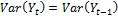).

However, some of the observed autocorrelation between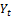and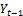were due to both being correlated with intervening lags. The PACF on the other hand seeks to measure the autocorrelation betweenandcorrecting for the correlation with intervening lags.

The log likelihood ratio test, AIC and the BIC were used for model diagnostic checks. Adequacy of the model was carried out for all cases through the analysis of the residuals by use of the Ljung-Pierce Q-statistics. In addition to the residual plots, the Maximum Likelihood Estimate (MSE) was used to check on the efficiency of the model. These were facilitated by use of Gretl statistical software.

Table 1. Data analysis matrix.

 Research hypothesis Independent Variable Dependent Variable Statistics Modeling and forecasting Kenyan GDP Time GDP Time plots, Correlogram, AIC, SBC, Log-likelihood, Cross tabulations, Least Squares, Durbin Watson , Students t

4. Main Results and Discussion

4.1. Basic Analysis

This study used a single set of data for Modeling that comprised of annual levels of GDP for Kenya. The data was obtained from the World Economic Outlook Database and the Kenya National Bureau of Statistics (KNBS) open data from 1960 to 2012. The preliminary analysis of the data was done by use of time plots for the series as shown by Figures 2 and 3 respectively.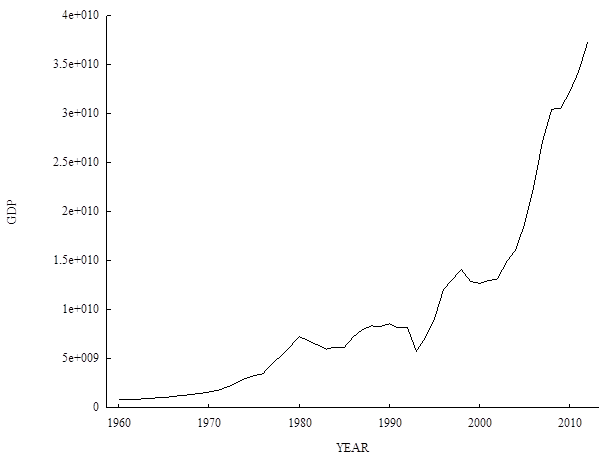Figure 2. Time plots for annual GDP levels.

From figure 1 above, a visual inspection of the time plots indicates that Kenyan GDP has shown the trend of exponential growth. This implies that both the mean and the variance are not constant. Therefore we regard it as a non-stationary time series.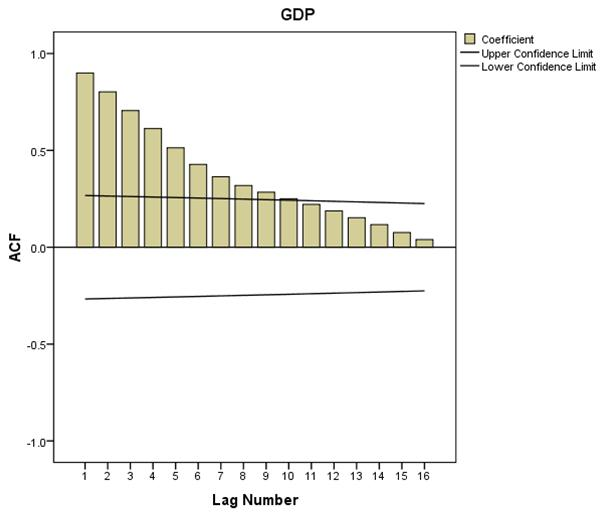Figure 3. Correlogram for Kenyan GDP data.

A visual examination of the correlogram above confirms that the Kenyan GDP data is non-stationary. This kind of non-stationary time series which contains a seasonal trend can often be carried out by logarithmic transformation. The result is that the exponential trend will be transformed into a linear trend. Before embarking on further analysis using the Box-Jenkins methodology the data has to be transformed to achieve stationarity.

The series was transformed by taking the second differences of the natural logarithms of the values in the series so as to attain stationarity in the second moment. The equation representing the transformation is given by;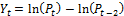where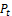represents the annual values for the series. The time plots for the returns are presented in figure 3.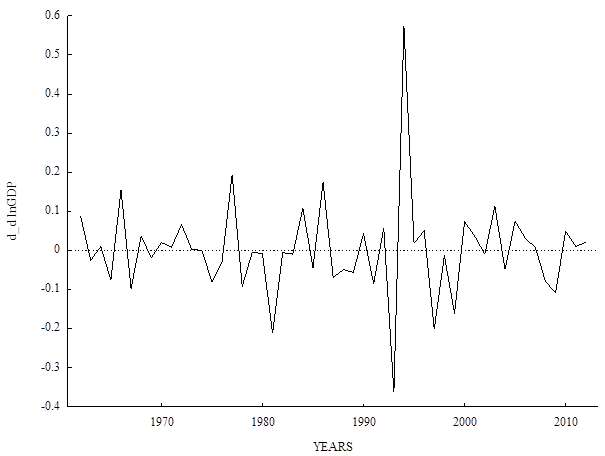Figure 4. Time plots for log differenced to degree two series.

4.2. Estimation Results

Modeling results of an ARIMA (2, 2, 2) process have been estimated by use of the Gaussian MLE Criterion and are presented in the table 2.

Table 2. Parameter estimates.

 Variable Estimate Std Error Z p-Value AR(1) -0.424586 0.228786 -1.856 0.0635 AR(2) 0.395116 0.130549 3.027 0.0025 MA(1) -0.265205 0.225381 -1.177 0.2393 MA(2) -0.734795 0.222153 -3.308 0.0009

4.2.1. Interpretation of the Estimation Results

The coefficient estimates of AR (1), AR (2), MA (1) and MA (2) schemes of Kenyan GDP shown in table 4, are statistically significant at 5 percent level of significance. Also, the estimates of AIC, SBC, Log likelihood and the Hannan-Quinn Criterion provide the minimum value hence implying a goodness of fit of the statistical model. Durbin-Watson statistic is near 2 indicating absence of both positive and negative autocorrelation.

4.2.2. Comparison with Other ARIMA Models

The above model was compared with different ARIMA models by use of model selection criteria such as Akaike information criterion, Log likelihood, Hannan-Quinn and Schwarz criterion, but the above model proved to be relatively robust compared to other competing models. The results are presented in table 3.

Table 3. Evaluation of various ARIMA models.

 ARIMA ARIMA ARIMA ARIMA ARIMA ARIMA (1, 2, 0) (1, 2, 1) (1, 2, 2) (2, 2, 0) (2, 2, 1) (2, 2, 2) Log Likelihood 38.0254 38.3674 43.0448 38.0413 38.69815 44.68355 Schwarz Criterion -68.1871 -64.9393 -70.3623 -64.2870 -61.6690 -69.70792 Alkaline criterion -72.0507 -70.7347 -78.0896 -70.0825 -69.3963 -79.36710 Hannan-Quinn -70.5743 -68.5201 -75.1368 -67.8679 -66.4435 -75.67606 SD of Innovations 0.1146 0.1138 0.10079 0.11458 0.11303 0.097601

The fitted ARIMA models were diagnosed using AIC, SBC and the log likelihood ratio test. Parameter estimation for the ARIMA models was done using the Gaussian MLE criterion. The ARIMA models fitted were adequate since the standardized residuals and squared residuals were not significantly correlated as shown by the Ljung-Box Q statistics. In addition, the J-B statistics strongly rejected the null hypothesis of normality in the residuals for all the series.

According to the results and evaluation of different ARIMA models as presented in tables 4 and 5 respectively, the best model can be re-written as follows: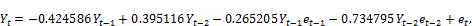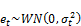(14)

Where;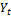represents the value of lnGDP.

From equation (14), basing on a 5 percent level of significance, it is clear that the observations are significant at the first lag and also the interaction between observations and the errors are significant at all the lags for the fitted model.

4.3. Out-of-Sample Forecasts

The study emphasized on forecast performance which suggests more focus on minimizing out-of-sample forecast errors than on maximizing in-sample goodness of fit. The approach adopted was therefore one of model mining with the objective of optimizing forecast performance.

The models efficiencies were evaluated using the Mean Squared Errors (MSE). The model that had the minimal MSE was considered the most efficient. However, other statistical properties especially the diagnostics and goodness of fit tests were considered in choosing the most efficient model. The MSE for the various ARMA models are given in table 4.

Table 4. The MSE of various ARMA Models.

 MODEL MSE ARIMA (0, 2, 0) 0.015648 ARIMA (0, 2, 1) 0.011664 ARIMA (0, 2, 2) 0.010904 ARIMA (1, 2, 0) 0.01316 ARIMA (1, 2, 1) 0.012972 ARIMA (1, 2, 2) 0.010607 ARIMA (2, 2, 0) 0.013152 ARIMA (2, 2, 1) 0.012804 ARIMA (2, 2, 2) 0.0099453

Considering the MSE values in Table 4 above, it is clear that ARIMA (2, 2, 2) model has the smallest value of the MSE thus the most efficient in Modeling and forecasting the Kenyan GDP. The chosen model therefore, is justified by its relatively lower values of residual Kurtosis and MSE in addition to the other diagnostics considered.

Therefore, other than within sample forecasts presented in appendix 1, the study also estimated five years out-of sample forecasts of the model to measure the forecasting ability. Results indicate that Kenyan GDP will continue to rise.

The forecasting power of the model is very high as indicated by the small difference between Actual and fitted values as presented in appendix 2. The five years ahead forecasts of Kenyan GDP are presented in table 5.

Table 5. Five Years ahead GDP Forecasts.

 Year lnGDP Forecast Std.Error GDP Forecast 2013 24.415792 0.097601 40146134650 2014 24.493442 0.160869 43387707470 2015 24.564373 0.222413 46577014250 2016 24.640186 0.270886 50245458410 2017 24.711272 0.316843 53947220100

5. Summary, Conclusion and Recommendations

5.1. Summary

The aim of the study was to model and forecast Kenyan GDP based on Box-Jenkins methodology and providing five years inflation forecasts of Kenya. Through collection and examination of the annual GDP data of Kenya, determining the order of integration, model identification, diagnostic checking, model stability testing, and forecast performance evaluation, the best ARIMA model was proposed in equation (14) based on the least mean squared error criteria. Time plots and the correlogram were used for testing stationarity of the data. Also, the Gaussian MLE Criterion was used for estimating the model.

5.2. Main Findings

The first main empirical finding of the study is the model that has been identified for forecasting GDP and it is presented below: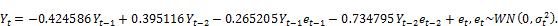(15)

Where:represents the value of lnGDP.

This is the forecasting model of GDP in Kenya that is recommended for consistent forecasting. All coefficients were statistically significant at 5 percent. Other statistical properties especially the diagnostics and goodness of fit tests were considered in choosing the most efficient model. Model efficiency was determined using the Mean Squared Error as shown in table 4.

Various ARIMA models with different order of Autoregressive and Moving Average terms were compared based on their performance, checked and verified by using the statistics such as AIC, SBC, Log-likelihood, Hannan Quinn Criterion and the Jarque-Bera statistic. The results indicate that the proposed model performed well in terms of both in-sample and out-of-sample.

The second empirical finding of the study is the 5 years GDP forecasts of Kenya. The out of sample short-run forecasts obtained indicate an increase in Kenyan GDP level.

5.3. Conclusion and Recommendation

Through time series analysis of Kenyan GDP in the years 1960 to 2007, the ARIMA (2, 2, 2) model was established. Transformation of the series by the model parameters turned the residual sequence into white noise sequence. The fitting result of the model is convincing and practical by using Gretl. The GDP of Kenya is forecasted by using the model.

The result shows that the relative error is within the range of 5%, which is relatively ideal. According to the values predicted, Kenyan GDP shows a higher growth trend in the next five years from 2013 to2017. However, the forecasting result of this model is only a predicted value; the national economy is a complex and dynamic system. The adjustments of macro policy and the changes of the development environment will cause the relative change of macro-economic indicators. Therefore, we should pay attention to the risk of adjustment in the economic operation and maintain the stability and continuity of the microeconomic regulation and control too prevent the economy from severe fluctuations and adjust the corresponding target value according to the actual situation.

5.4. Suggestions for Further Research

From the findings of the study, the following areas are suggested for further research:

i. Analysis of GDP Dynamics in Kenya using different models.

ii. Examination of individual components of the GDP.

Appendices

Appendix 1

Standard error of residuals = 0.0976013

Table 6. In-Sample GDP Forecasts 1960-2012.

 Year Actual GDP Actual lnGDP Fitted lnGDP 1962 868111400 20.5818 20.4934 1963 926589400 20.6470 20.6322 1964 998759400 20.7220 20.7166 1965 997919300 20.7212 20.7738 1966 1164520000 20.8756 20.7596 1967 1232559000 20.9324 20.9512 1968 1353295000 21.0258 21.0218 1969 1458379000 21.1006 21.0767 1970 1603447000 21.1954 21.1911 1971 1778391000 21.2990 21.2577 1972 2107279000 21.4687 21.3961 1973 2502142000 21.6404 21.5719 1974 2973309000 21.8129 21.7746 1975 3259345000 21.9048 21.9313 1976 3474542000 21.9687 22.0103 1977 4494379000 22.2261 22.0396 1978 5303735000 22.3917 22.3797 1979 6234391000 22.5533 22.5405 1980 7265315000 22.7064 22.6693 1981 6854492000 22.6482 22.8444 1982 6431579000 22.5845 22.6936 1983 5979198000 22.5116 22.6018 1984 6191437000 22.5464 22.5374 1985 6135034000 22.5373 22.5934 1986 7239127000 22.7028 22.5958 1987 7970821000 22.7991 22.7919 1988 8355381000 22.8462 22.9164 1989 8283114000 22.8375 22.8980 1990 8572359000 22.8718 22.8970 1991 8151489000 22.8215 22.9147 1992 8209121000 22.8285 22.8638 1993 5751786000 22.4728 22.8525 1994 7148149000 22.6901 22.4086 1995 9046320000 22.9256 22.7250 1996 12045860000 23.2120 23.1311 1997 13115760000 23.2971 23.3212 1998 14094000000 23.3690 23.4357 1999 12896010000 23.2802 23.4003 2000 12705350000 23.2653 23.3310 2001 12985990000 23.2871 23.2574 2002 13147740000 23.2995 23.3626 2003 14904500000 23.4249 23.3244 2004 16095320000 23.5018 23.5195 2005 18737900000 23.6538 23.5760 2006 22504140000 23.8370 23.7485 2007 27236740000 24.0278 23.9590 2008 30465490000 24.1399 24.1472 2009 30580370000 24.1436 24.2407 2010 32230612377 24.1962 24.1913 2011 34329924186 24.2593 24.2540 2012 37338072592 24.3433 24.3324

Appendix 2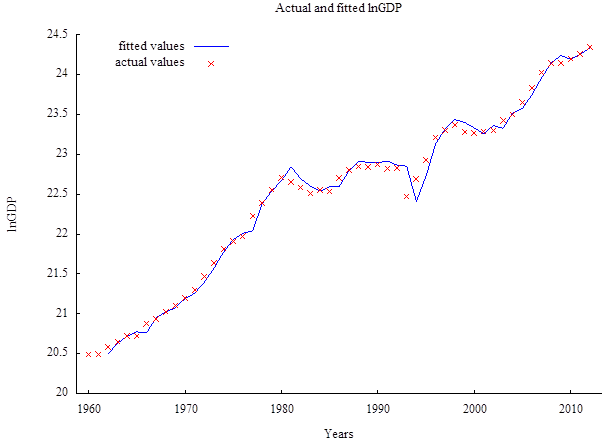Figure 5. Time Plot for Actual and Fitted lnGDP values.

References

1. Ahmad, J. and Harnhirun, S. (1996), Cointegration and causality between exports and economic growth: evidence from the Asian countries. Canadian Journal of Economics, 413-6.
2. Ard, H. J, den Reijer. (2010), Macroeconomic Forecasting using Business Cycle leading indicators, Stockholm: US-AB.
3. Barro, R. J. (1990). Economic Growth in a Cross section of Countries. The Quarterly Journal of Economics, 47-53.
4. Box, G. E. P., and Jenkins, G., (1970). Time Series Analysis, Forecasting and Control, Holden-Day, San Francisco.
5. Box, George E. P. and Gwilym M. Jenkins (1976). Time Series Analysis: Forecasting and Control, Revised Edition, Oakland, CA: Holden-Day.
6. Cheng, Ming-yu, T. Hui-Boon (2002), Faculty of Management, Multimedia University,Malaysia, Journal of Inflation in Malaysia, 29(5), 411-425.
7. Chatfield, C. (1996). The Analysis of Time Series, 5th ed., Chapman & Hall, New York, NY.
8. Dodaro, S. (1993). Exports and Growth: A Reconsideration of causity. Journal of Developing Areas, 227-234.
9. Dullah, L. and Kasim. (2010). Determinant factors of Economic growth in Malaysia: Multivariate cointegration and Causality analysis. European Journal of Economics, Finance and Administrative Sciences, ISSN, 1450-2275.
10. Hayek, Friedrich (1989). The Collected Works of F. A. Hayek. University of Chicago Press. p. 202. ISBN 978-0-226-32097-7.
11. Heller, P. S, and R. C Porter. (1978). "Exports and growth: An Empirical Investigation." Journal of Development Economics, 191-193.
12. Leedy, P. D. (1997). Practical research planning and design (6th ed.). Upper Saddle River, NJ: Prentice-Hall, Inc., 232-233.
13. Litterman, R. B. (1986). Forecasting with Bayesian Vector Autoregressions-five Years of experience. Journal of Business & Economic Statistics, 25-38.
14. Mugenda O. M. and Mugenda A. G. (2003). Research Methods: Quantitative and Qualitative Approaches. Nairobi, Kenya. Acts Press.
15. Ning, W., Kuan-jiang, B. and Zhi-fa, Y. (2010), Analysis and forecast of Shaanxi GDP based on the ARIMA Model, Asian Agricultural Research, Vol. 2 No. 1, pp. 34-41.
16. Ram, R. (1986). Government Size and Economic Growth.A new Framework and some Empirical Evidence from Cross-sectional and Time Series Data, 191-203.
17. Sheehey, Edmund J., (1992), ‘Exports and Growth: Additional Evidence’, Journal of Development Studies, Vol.28, No.4.
18. Sinha, D. and Sinha, T., (2007), Toda and Yamamoto causality test between per capita saving and per capita GDP for India, MRPA Paper No 2564.
19. Slutzky Eugen, (Apr., 1937), The Summation of Random Causes as the Source of Cyclic Processes, Econometrica, Vol. 5,No. 2, pp. 105-146.
20. Stockton, David J. and James E. Glassman (1987). "An Evaluation of the Forecast Performance of Alternative Models of Inflation," The Review of Economics and Statistics 69, 108-117.
21. Wold H. (1938), A Study in Analysis of Stationary Time Series, Uppsala.
22. Yoo J, Maddala. (1991). Risk Premia and Price Volatility in Future Markets. Journal of Future Markets, 11 (2): 165-177.
23. Yule G. U., (Jan., 1926). Why do we sometimes get Nonsense-Correlations between Time-Series? A Study in Sampling and the Nature of Time-Series, Journal of the Royal Statistical Society, Vol. 89, No. 1, pp. 1-63.

 Contents 1. 2. 2.1. 2.2. 2.3. 3. 3.1. 3.2. 3.3. 3.4. 3.5. 4. 4.1. 4.2. 4.3. 5. 5.1. 5.2. 5.3. 5.4.
Article ToolsAbstractPDF(531K)